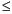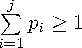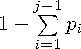Functions and CALL Routines

# RANTBL Function

Returns a random variate from a tabled probability distribution.
 Category: Random Number Tip: If you want to change the seed value during execution, you must use the CALL RANTBL routine instead of the RANTBL function.

## Syntax

 RANTBL(seed,p1 ,... pi... ,pn)

### Arguments

seed

is a numeric constant, variable, or expression with an integer value. If seed0, the time of day is used to initialize the seed stream.

pi

is a numeric constant, variable, or expression.

 Range: 0pi1 for 0

The RANTBL function returns a variate that is generated from the probability mass function defined by p1 through pn . An inverse transform method applied to a RANUNI uniform variate is used. RANTBL returnsIf, for some index j<n,, RANTBL returns only the indices 1 through j with the probability of occurrence of the index j equal to.

Let n=3 and P1, P2, and P3 be three probabilities with P1+P2+P3=1, and M1, M2, and M3 be three variables. The variable X in these statements

```array m{3} m1-m3;
x=m{rantbl(seed,of p1-p3)};```

will be assigned one of the values of M1, M2, or M3 with probabilities of occurrence P1, P2, and P3, respectively.

For a discussion and example of an effective use of the random number CALL routines, see Starting, Stopping, and Restarting a Stream.

The CALL RANTBL routine, an alternative to the RANTBL function, gives greater control of the seed and random number streams.

 Functions and CALL routines:Previous Page | Next Page | Top of Page